# AttributeError: 'list' object has no attribute 'shape'Last updated: Feb 2, 2023
5 min## #AttributeError: 'list' object has no attribute 'shape'

The Python "AttributeError: 'list' object has no attribute 'shape'" occurs when we try to access the `shape` attribute on a list.

To solve the error, pass the list to the `numpy.array()` method to create a numpy array before accessing the `shape` attribute.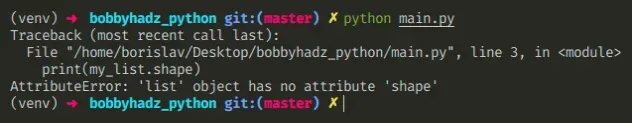Here is an example of how the error occurs.

main.py
```Copied!```my_list = [1, 2, 3]

# # ⛔️ AttributeError: 'list' object has no attribute 'shape'
print(my_list.shape)
``````

Native Python lists don't have a `shape` attribute, instead you have to convert the list to a NumPy array.

## #Convert the list to a NumPy array

To solve the error, pass the list to the `np.array()` method to create a numpy array.

main.py
```Copied!```import numpy as np

np_array = np.array([1, 2, 3])

print(np_array.shape)  # 👉️ (3,)
``````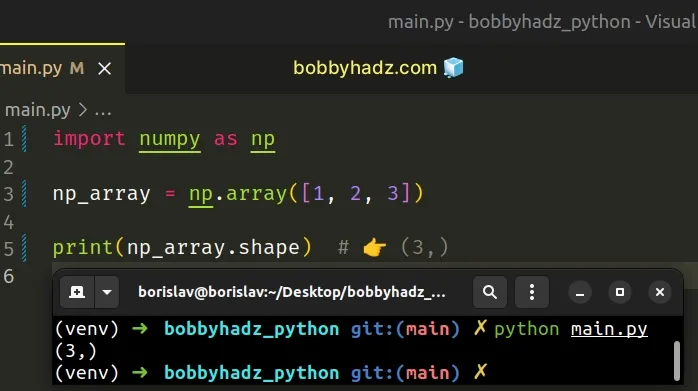The numpy.array method takes an array-like object as an argument and creates an array.

You might also use the numpy.asarray method to convert a list to a NumPy array before accessing the `shape` attribute.

main.py
```Copied!```import numpy as np

np_array = np.asarray([1, 2, 3])

print(np_array.shape)  # 👉️ (3,)
``````

## #Passing the list directly to the shape() method

Alternatively, you can pass the list directly to the numpy.shape method.

main.py
```Copied!```import numpy as np

array_shape = np.shape([1, 2, 3])
print(array_shape)  # 👉️ (3,)

print(np.shape([[1, 2]]))  # 👉️ (1, 2)

print(np.shape())  # 👉️ (1,)
``````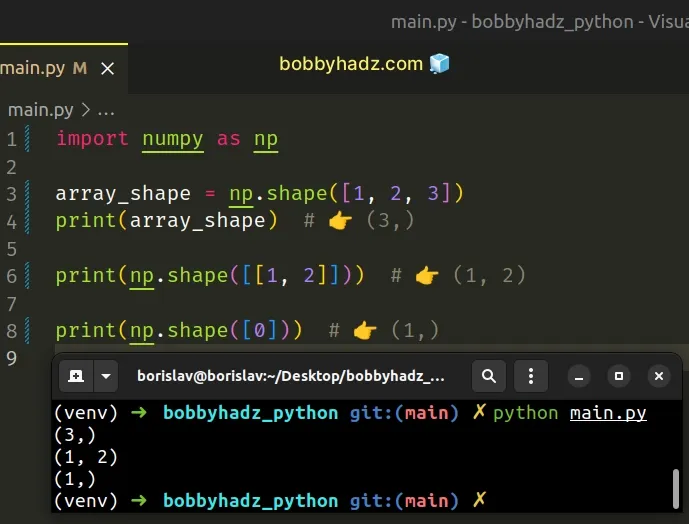The `numpy.shape` method takes an array-like object as an argument and returns the shape of the array.

The elements of the `shape` tuple give the lengths of the corresponding array dimensions.

## #Getting the length of a list

If you need to get the length of a list, use the `len()` function.

main.py
```Copied!```my_list = [[1, 2, 3], [4, 5, 6]]

# 👇️ get length of entire list
print(len(my_list))  # 👉️ 2

# 👇️ get length of list item at index 0
print(len(my_list))  # 👉️ 3
``````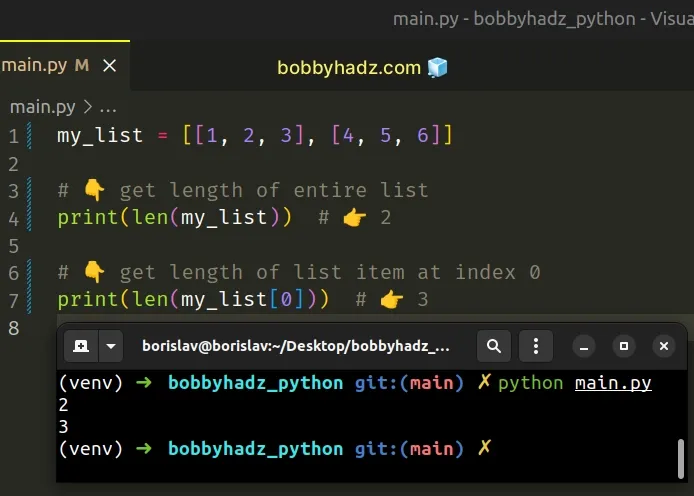The len() function returns the length (the number of items) of an object.

The argument the function takes may be a sequence (a string, tuple, list, range or bytes) or a collection (a dictionary, set, or frozen set).

## #Checking all of the attributes of an object

You can view all the attributes an object has by using the `dir()` function.

main.py
```Copied!```my_list = ['bobby', 'hadz', 'com']

# 👉️ [... 'append', 'clear', 'copy', 'count', 'extend', 'index',
#  'insert', 'pop', 'remove', 'reverse', 'sort' ...]
print(dir(my_list))
``````

If you pass a class to the dir() function, it returns a list of names of the class's attributes, and recursively of the attributes of its bases.

If you try to access any attribute that is not in this list, you would get the "AttributeError: list object has no attribute" error.

## #AttributeError: 'list' object has no attribute 'reshape'

The Python "AttributeError: 'list' object has no attribute 'reshape'" occurs when we try to call the `reshape()` method on a list.

To solve the error, pass the list to the `numpy.array()` method to create a numpy array before calling the `reshape` method.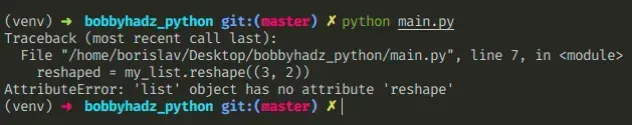Here is an example of how the error occurs.

main.py
```Copied!```my_list = [0, 1, 2, 3, 4, 5]

# ⛔️ AttributeError: 'list' object has no attribute 'reshape'
reshaped = my_list.reshape((3, 2))
``````

Python lists don't have a `reshape()` attribute. To solve the error, convert the list to a NumPy array.

## #Convert the list to an array before calling reshape()

To solve the error, pass the list to the `np.array()` method to create a `numpy` array.

main.py
```Copied!```import numpy as np

my_list = [0, 1, 2, 3, 4, 5]

np_array = np.array(my_list)

# [[0 1]
#  [2 3]
#  [4 5]]
print(np_array.reshape((3, 2)))
``````

The numpy.array method takes an array-like object as an argument and creates an array.

You might also use the numpy.asarray method to convert a list into a `numpy` array before calling the `reshape` method.

main.py
```Copied!```import numpy as np

my_list = [0, 1, 2, 3, 4, 5]

np_array = np.asarray(my_list)

# [[0 1]
#  [2 3]
#  [4 5]]
print(np_array.reshape((3, 2)))
``````

## #Passing the list directly to the numpy.reshape() method

Alternatively, you can pass the list directly to the numpy.reshape method.

main.py
```Copied!```import numpy as np

my_list = [0, 1, 2, 3, 4, 5]

reshaped = np.reshape(my_list, (3, 2))

# [[0 1]
#  [2 3]
#  [4 5]]
print(reshaped)
``````

The `numpy.reshape` method gives a new shape to an array without changing its data.

main.py
```Copied!```import numpy as np

my_list = [[0, 1, 2], [3, 4, 5]]

reshaped = np.reshape(my_list, 6)

print(reshaped)  # [0 1 2 3 4 5]
``````

The `np.reshape` method takes an array-like object as an argument and an int or tuple of integers that represent the new shape.

The new shape should be compatible with the original shape.

If an integer is passed for the new shape argument, the result will be a 1-D array of that length.

If you need to get the length of a list, use the `len()` function.

main.py
```Copied!```my_list = [[1, 2, 3], [4, 5, 6]]

# 👇️ get length of entire list
print(len(my_list))  # 👉️ 2

# 👇️ get length of list item at index 0
print(len(my_list))  # 👉️ 3
``````

The len() function returns the length (the number of items) of an object.

The argument the function takes may be a sequence (a string, tuple, list, range or bytes) or a collection (a dictionary, set, or frozen set).

You can view all the attributes an object has by using the `dir()` function.

main.py
```Copied!```my_list = ['bobby', 'hadz', 'com']

# 👉️ [... 'append', 'clear', 'copy', 'count', 'extend', 'index',
#  'insert', 'pop', 'remove', 'reverse', 'sort' ...]
print(dir(my_list))
``````

If you pass a class to the dir() function, it returns a list of names of the class's attributes, and recursively of the attributes of its bases.

If you try to access any attribute that is not in this list, you would get the "AttributeError: list object has no attribute" error.

## #AttributeError: 'list' object has no attribute 'astype'

The Python "AttributeError: 'list' object has no attribute 'astype'" occurs when we try to call the `astype()` method on a list.

To solve the error, pass the list to the `numpy.array()` method to create a numpy array before calling the `astype` method.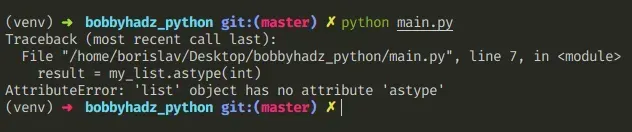Here is an example of how the error occurs.

main.py
```Copied!```my_list = [1, 2, 2.5, 3.6]

# ⛔️ AttributeError: 'list' object has no attribute 'astype'
result = my_list.astype(int)
``````

Native Python lists don't have an `astype` attribute.

To solve the error, we have to convert the list to a NumPy array.

## #Convert the list to an array before using astype()

To solve the error, pass the list to the `np.array()` method to create a NumPy array.

main.py
```Copied!```import numpy as np

my_list = [1, 2, 2.5, 3.6]

np_array = np.array(my_list, dtype=np.int32)

print(np_array)  # 👉️ [1 2 2 3]
``````

The dtype keyword argument can be used to set the data type of the array's elements.

You can use the astype method on a NumPy array to copy the array and cast it to a specified type.

main.py
```Copied!```import numpy as np

my_list = [1, 2, 2.5, 3.6]

np_array = np.array(my_list, dtype=np.int32)

print(np_array)  # 👉️ [1 2 2 3]

result = np_array.astype(np.float32)

print(result)  # 👉️ [1. 2. 2. 3.]
``````

The only parameter we passed to the `astype` method is the data type to which the array is cast.

main.py
```Copied!```import numpy as np

my_list = [1, 2, 2.5, 3.6]

np_array = np.array(my_list)

result = np_array.astype(int)

print(result)  # 👉️ [1 2 2 3]
``````

You can view all the attributes an object has by using the `dir()` function.

main.py
```Copied!```my_list = ['a', 'b', 'c']

# 👉️ [... 'append', 'clear', 'copy', 'count', 'extend', 'index',
#  'insert', 'pop', 'remove', 'reverse', 'sort' ...]
print(dir(my_list))
``````

If you pass a class to the dir() function, it returns a list of names of the class's attributes, and recursively of the attributes of its bases.

If you try to access any attribute that is not in this list, you would get the "AttributeError: list object has no attribute" error.

I wrote a book in which I share everything I know about how to become a better, more efficient programmer.You can use the search field on my Home Page to filter through all of my articles.